### Home > PC > Chapter 2 > Lesson 2.3.5 > Problem2-134

2-134.
1. Write each rational expression as a single simplified fraction. Homework Help ✎

1.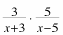2.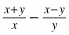3.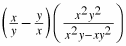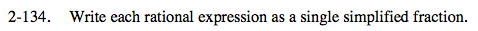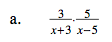Multiply numerator with numerator and denominator with denominator.
Then simply if possible.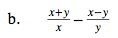$\text{Mutiply the first fraction by }\frac{y}{y}\text{ and the second fraction by }\frac{x}{x},$

$\text{giving you a common denominator of }xy.$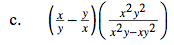Simplify the expression in the first set of parentheses by creating a common denominator. Then multiply.